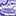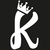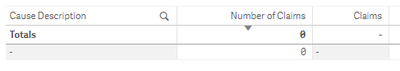# New to Qlik Sense

If you’re new to Qlik Sense, start with this Discussion Board and get up-to-speed quickly.

Announcements
WE ARE LISTENING! New Navigation for Qlik Community, Sept. 26: TELL ME MORE
cancel
Showing results for
Did you mean:Contributor III

## % of total on top 10 table

Hi All

i have created a table in Qlik that, when fully displayed, looks something like the below.

 CAUSEDESCRIPTION CLAIMNO Insured 1 177 Insured 2 145 Insured 3 81 Insured 4 78 Insured 5 45 Insured 6 45 Insured 7 27 Insured 8 19 Insured 9 18 Insured 10 13 Insured 11 8 Insured 12 5 Insured 13 3 TOTAL 664

I wanted to show the top 10 causes and include the count of CLAIMNO, i set to top 10 in the dimension. it displayed the correct number of fields and with the correct count of CLAIMNO assigned to each of the top 10 CAUSEDESCRIPTION filed with the exception of the column total. this still showed 664 even through if you were to add up when was displayed it would sum to 648.

To get around this i found i need to do the following dimension

IF(Aggr(Rank(SUM(CLAIMNO),4),CAUSEDESCRIPTION)<=10,CAUSEDESCRIPTION)

this now shows everything as i expect. Great! i then wanted to know what each of the top 10 CAUSEDESCRIPTION % was in comparison to the total of the top 10. i.e. Insured 1 would calc as 177/648. i had expected to merely do count(CLAIMNO)/Count(Total CLAIMNO) but this is calculating with 177/664.

so my actual question is how do i do the calculation so it uses the 648 as the division rather than the full table total?

thanks

1 Solution

Accepted SolutionsPartner - Specialist

Hi David,

For Top10: You can use this Expression

Count({<ProductName = {"=Rank(Count(OrderID))<=10"} >}OrderID)

For Total of Top10 :

Count(Total {<ProductName = {"=Rank(Count(OrderID))<=10"} >}OrderID)

For percentage Calculation:

Count({<ProductName = {"=Rank(Count(OrderID))<=10"} >}OrderID)
/ Count(Total {<ProductName = {"=Rank(Sum(Quantity))<=10"} >}OrderID)

output is attached

Hope it works for you

Thanks and Regards
Kashyap.R
5 RepliesCreator III

Not sure if I got that right, but try this:

Instead of that expression as dimension, use the if inside the aggr():

Aggr( if(Rank(Sum( Total CLAIMNO))<=10, CAUSEDESCRIPTION), CAUSEDESCRIPTION)

and then, as measuse, try the Count(CLAIMNO) as usual, otherwise try

Aggr( if(Rank(Sum( Total CLAIMNO))<=10, Count(CLAIMNO)), CAUSEDESCRIPTION)

Hope it helpsContributor III
Author
Aviva: Public

Thank but although Qlik does accept the dimension as correct it unfortunately this clears all fields to blanks or nulls when I put your dimension in place and count(CLAIMNO)
[cid:image001.png@01D56169.5A25E170]Contributor III
Author

Thank but although Qlik does accept the dimension as correct it unfortunately then clears all fields to blanks or nulls when I put your dimension in place and try either count(CLAIMNO) or Aggr( if(Rank(Sum( Total CLAIMNO))<=10, Count(CLAIMNO)), CAUSEDESCRIPTION).Partner - Specialist

Hi David,

For Top10: You can use this Expression

Count({<ProductName = {"=Rank(Count(OrderID))<=10"} >}OrderID)

For Total of Top10 :

Count(Total {<ProductName = {"=Rank(Count(OrderID))<=10"} >}OrderID)

For percentage Calculation:

Count({<ProductName = {"=Rank(Count(OrderID))<=10"} >}OrderID)
/ Count(Total {<ProductName = {"=Rank(Sum(Quantity))<=10"} >}OrderID)

output is attached

Hope it works for you

Thanks and Regards
Kashyap.RContributor III
Author

worked perfectly. thank you